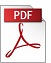### “Using Generalized Fibonacci Sequences for Solving the One-Dimensional LQR Problem and its Discrete-Time Riccati Equation”

Authors: Johan Byström, Lars P. Lystad and Per-Ole Nyman,
Affiliation: Luleå University of Technology and Narvik University College
Reference: 2010, Vol 31, No 1, pp. 1-18.

Keywords: LQR, Linear quadratic control, Optimal control, Fibonacci number, Golden ratio, Binet formula

Abstract: In this article we develop a method of solving general one-dimensional Linear Quadratic Regulator (LQR) problems in optimal control theory, using a generalized form of Fibonacci numbers. We find the solution R(k) of the corresponding discrete-time Riccati equation in terms of ratios of generalized Fibonacci numbers. An explicit Binet type formula for R(k) is also found, removing the need for recursively finding the solution at a given timestep. Moreover, we show that it is also possible to express the feedback gain, the penalty functional and the controller state in terms of these ratios. A generalized golden ratio appears in the corresponding infinite horizon problem. Finally, we show the use of the method in a few examples.PDF (430 Kb)        DOI: 10.4173/mic.2010.1.1

  Thomas Brasch, Johan Byström and Lars Petter Lystad (2012), doi:10.1007/s10957-012-0061-2  Zhen Chen, Binglong Cong and Xiangdong Liu (2013), doi:10.1155/2013/602869  Przemys aw Ignaciuk (2016), doi:10.1016/j.jfranklin.2015.03.033
References:
 Balchen, J. (1977). Reguleringsteknikk, Tapir, Trondheim.
 Benavoli, A., Chisci, L., Farina, A. (2009). Fibonacci sequence, golden section, Kalman filter and optimal control, Signal Processing, 8.8:1483--1488 doi:10.1016/j.sigpro.2009.02.003
 Camouzis, E. Ladas, G. (2008). Dynamics of Third Order Rational Difference Equations with Open Problems and Conjectures, Chapman and&amp; Hall / CRC, Boca Raton.
 Capponi, A., Farina, A., Pilotto, C. (2010). Expressing stochastic filters via number sequences, Signal Processing, 9.7:2124--2132 doi:10.1016/j.sigpro.2010.01.011
 Dickson, L.E. (2002). History of the Theory of Numbers, Volume I: Divisibility and Primality, The American Mathematical Society.
 Dunlap, R. (2003). The Golden Ratio and Fibonacci Numbers, World Scientific, Singapore.
 Elaydi, S. (2005). An Introduction to Difference Equations, 3rd ed.. Springer, New York.
 Huntley, H.E. (1970). The Divine Proportion: a Study in Mathematical Beauty, Dover Publications, Mineola.
 Kulenovic, M. R.S. Ladas, G. (2002). Dynamics of Second Order Rational Difference Equations: With open problems and conjectures, Chapman and&amp; Hall / CRC, Boca Raton.
 Kwakernaak, H. Sivan, R. (1972). Linear optimal control systems, Wiley-Interscience, New York-London-Sydney.
 Lang, W. (2004). Riccati meets fibonacci, The Fibonacci Quarterly, 4.3:231--244.
 Lewis, F.L. Syrmos, V.L. (1995). Optimal control, 2nd Ed.. John Wiley and&amp; Sons Inc., New York.
 Livio, M. (2002). The Golden Ratio: The Story of PHI, the World´s Most Astonishing Number, Broadway Books.
 Ribenboim, P. (2000). My Numbers, My Friends: Popular Lectures on Number Theory, Springer Verlag, New York.
 Walser, H. (2001). The Golden Section, The Mathematical Association of America.
 Åström, K. Wittenmark, B. (1996). Computer-Controlled Systems: Theory and Design, Prentice Hall.

BibTeX:
@article{MIC-2010-1-1,
title={{Using Generalized Fibonacci Sequences for Solving the One-Dimensional LQR Problem and its Discrete-Time Riccati Equation}},
author={Byström, Johan and Lystad, Lars P. and Nyman, Per-Ole},
journal={Modeling, Identification and Control},
volume={31},
number={1},
pages={1--18},
year={2010},
doi={10.4173/mic.2010.1.1},
publisher={Norwegian Society of Automatic Control}
};